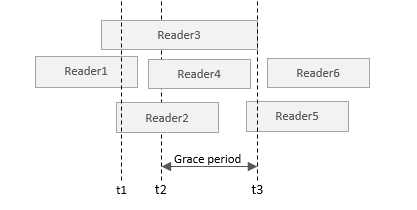# 深入理解 Linux 的 RCU 机制

2019年6月18日 作者 oceansw

RCU(Read-Copy Update)，是 Linux 中比较重要的一种同步机制。顾名思义就是“读，拷贝更新”，再直白点是“随意读，但更新数据的时候，需要先复制一份副本，在副本上完成修改，再一次性地替换旧数据”。这是 Linux 内核实现的一种针对“读多写少”的共享数据的同步机制。

RCU 的一个典型的应用场景是链表，在 Linux kernel 中还专门提供了一个头文件（include/linux/rculist.h），提供了利用 RCU 机制对链表进行增删查改操作的接口。本文将通过一个例子，利用 rculist.h 提供的接口对链表进行增删查改的操作，来讲述 RCU 的原理，以及介绍 Linux kernel 中相关的 API（基于 Linux v3.4.0 的源码）。

## 增加链表项

Linux kernel 中利用 RCU 往链表增加项的源码如下：

```#define list_next_rcu(list)     (*((struct list_head __rcu **)(&(list)->next)))

{
new->next = next;
new->prev = prev;
rcu_assign_pointer(list_next_rcu(prev), new);
next->prev = new;
}```

list_next_rcu() 函数中的 rcu 是一个供代码分析工具 Sparse 使用的编译选项，规定有 rcu 标签的指针不能直接使用，而需要使用 rcu_dereference() 返回一个受 RCU 保护的指针才能使用。rcu_dereference() 接口的相关知识会在后文介绍，这一节重点关注 rcu_assign_pointer() 接口。首先看一下 rcu_assign_pointer() 的源码：

```#define __rcu_assign_pointer(p, v, space) \
({ \
smp_wmb(); \
(p) = (typeof(*v) __force space *)(v); \
})```

## 访问链表项

Linux kernel 中访问 RCU 链表项常见的代码模式是：

```rcu_read_lock();
// do something with `pos`
}

```#define __rcu_dereference_check(p, c, space) \
({ \
typeof(*p) *_________p1 = (typeof(*p)*__force )ACCESS_ONCE(p); \
rcu_lockdep_assert(c, "suspicious rcu_dereference_check()" \
" usage"); \
rcu_dereference_sparse(p, space); \
((typeof(*p) __force __kernel *)(_________p1)); \
})

```rcu_read_lock();
p1 = rcu_dereference(p);
if (p1 != NULL) {
// do something with p1, such as:
printk("%d\n", p1->field);
}

```/* `p` 指向一块受 RCU 保护的共享数据 */

p1 = rcu_dereference(p);
if (p1 != NULL) {
printk("%d\n", p1->field);
}

/* free the memory */
p2 = p;
if (p2 != NULL) {
p = NULL;
synchronize_rcu();
kfree(p2);
}```## 删除链表项

```p = seach_the_entry_to_delete();
list_del_rcu(p->list);
synchronize_rcu();
kfree(p);

/* list.h */
{
next->prev = prev;
prev->next = next;
}

/* rculist.h */
static inline void list_del_rcu(struct list_head *entry)
{
__list_del(entry->prev, entry->next);
entry->prev = LIST_POISON2;
}```

## 更新链表项

```p = search_the_entry_to_update();
q = kmalloc(sizeof(*p), GFP_KERNEL);
*q = *p;
q->field = new_value;
list_replace_rcu(&p->list, &q->list);
synchronize_rcu();
kfree(p);```

```static inline void list_replace_rcu(struct list_head *old,